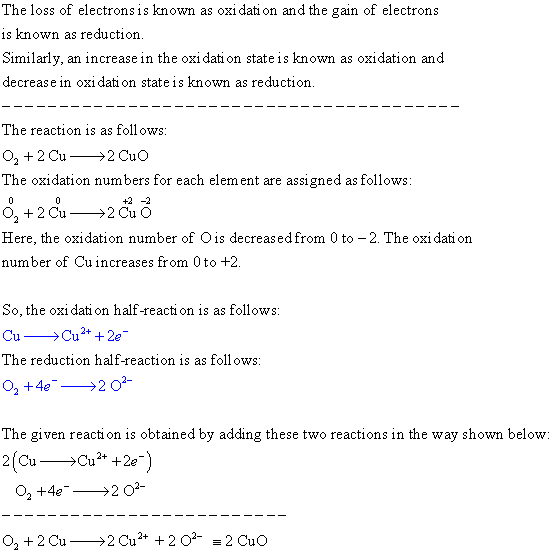# Separate this redox reaction into its component half reactions

Separate this redox reaction into its component
half-reactions. O2 + 2Cu –> 2CuO
2

The loss of electrons is known as oxi dati on and the gain of electrons is known as reduction Similarly, an increase in the oxi dati on state is known as oxidation and decrease in oxi dati on state is known as reduction The reaction is as follows: The oxidation numbers for each element are assigned as follows: 02 +2 Cu2 Cu O Here, the oxidation number of O is decreased from 0 to -2. The oxi dati on number of Cu increases from 0 to +2 So, the oxti dation half reaction is as follows The reduction half-reaction is as foll ows O2 +4e-→202- The given reacti on is obtained by adding these two reactions in the way shown below: 2(Cu Cu 2e 02 +4e2 O O22Cu2 Cu2 202 CuO